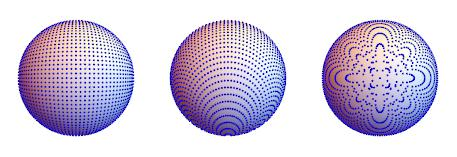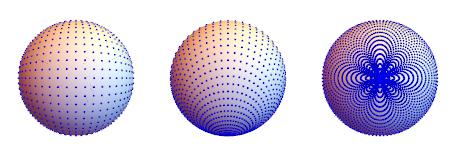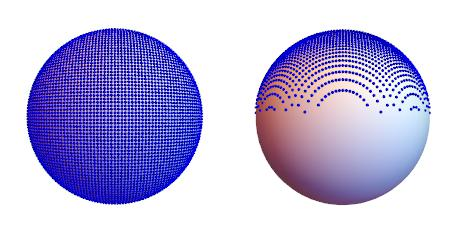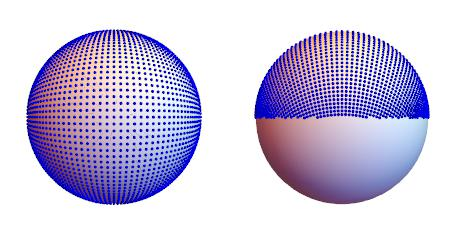This was part of a draft version of “Quaternion half/double angle and Cayley transforms”. I broke this out because the methods are indepenent of either and are simply implications of computing relative quaternions and using the similarity transform.

We can rotate a bivector with the quaternion similarity transform. Given unit quaternion $Q$ then the rotation of $a$ to $b$ can be expressed as:

$\mathbf{b} = Q~\mathbf{a}~Q^*$

When $a$ and $b$ are unit bivectors then solving for $Q$ yields:

$\begin{equation} \label{xform} Q = \sqrt{\mathbf{ba}^*} \end{equation}$

Using the previous and a known $a$ we can implictly represent $b$ by $Q$. Since we have bivector inputs $\eqref{xform}$ can be reduced:

$\begin{eqnarray*} Q &=& \sqrt{\mathbf{ba}^*} \\ &=& \sqrt{-\mathbf{ba}} \\ &=& \sqrt{a \cdot b + a \times b} \end{eqnarray*}$

Breaking down these two steps:

• Extract the relative angle information of $b$ with respect to $a$ is: $ba^*$. This can also be expressed as: $ba^{-1}$. The difference is the former composes the scale and the later computes relative scale. These hold for quaternions in general.

• Once we have the relative information applying the half-angle transform (square root) completes the forward transform.

I will only talk about unit bivectors but the same holds for arbitrary magnitude the math just needs to be carried through for that case.

Notice that the action here is really two dimensional. The first products’ role to to change our angle measuring stick from the direction of positive reals to the direction of $a$. It simply maps a unit sphere to a unit sphere. The square root is a complex valued function so the action is in 2D: $\mathbf{ba}^*$ and $\sqrt{\mathbf{ba}^*}$ are in the sample complex plane, the plane that spans {$1, a\times b$}.

## Example: let $b$ be up (as in positive $z$)

As an example we can create a context free representation of normals by choosing a reference direction like positive $z$. Renaming $\mathbf{a}$ to $\mathbf{z}$ and $\mathbf{b}$ to $\mathbf{n}$ with:

$\begin{eqnarray*} \mathbf{n} &=& \left(x,~y,~z\right) \\ \mathbf{z} &=& \left(0,~0,~1\right) \end{eqnarray*}$

then $Q$ can be expressed as:

$\begin{eqnarray} Q & = & \sqrt{\mathbf{n}\mathbf{z}^*} \nonumber \\ & = & \sqrt{z + \left(-y,~x,~0 \right)} \nonumber \\ & = & \frac{1+z}{\sqrt{2+2z}} + \left(\frac{-y}{\sqrt{2+2z}},~\frac{x}{\sqrt{2+2z}},~0\right) \label{fx} \end{eqnarray}$

Renaming this result as:

$Q = a + \left(-b,~c,~0\right)$

When $\mathbf{n}=-\mathbf{z}$ the equation explodes but the result is not infinity. We have $\sqrt{-1}$ which generally for quaternions means “any unit bivector” so $a=0$ and $(-b)^2+c^2=1$. Following through with $Q$ is a rotation that maps $\mathbf{z}$ to $-\mathbf{z}$ leads to the same result.

At this point we have all the constraints on the values as listed at the end of the Preliminary stuff section of the half-angle/Cayley post1. Additionally we have the bivector part is orthogonal to the reference direction.

The reverse transform becomes:

$\begin{eqnarray} n & = & Q~\mathbf{z}~Q^* \nonumber \\ & = & \left(2ac,~2ab,~a^2-b^2-c^2\right) \nonumber \\ & = & \left(2ac,~2ab,~1-2\left(b^2+c^2\right)\right) \label{rx1} \\ & = & \left(2c\sqrt{1-\left(b^2+c^2\right)},~2b\sqrt{1-\left(b^2+c^2\right)},~1-2\left(b^2+c^2\right)\right) \label{rx2} \end{eqnarray}$

where $\eqref{rx1}$ is one way to reduce if we have all three values and $\eqref{rx2}$ is reworked for reconstructing from the orthogonal projection.

We can visualize the forward transform as operations on a globe in its original space. The north pole $\mathbf{z}$ is the fixed point. We poke a hole at the south pole $-\mathbf{z}$ (breaking it into an infinite number of copies) and pull it to the equator which now contains the infinite copies of it. All other points on the sphere remain on the same longitude and the angle measure of each with respect to $\mathbf{z}$ is halved.

If we are projecting into the disc, then we view this global looking straight at the north pole using an orthographic projection. This disc projection is an area-preserving map.

• the north pole is mapped to $\left( 0, ~0 \right)$
• positive half sphere $\left( \mathbf{z} \geq 0 \right)$ is mapped to the disc: $r \in \left[ 0, ~\frac{1}{\sqrt{2}} \right]$
• negative half sphere $\left( \mathbf{z} < 0 \right )$ is mapped to the annulus: $r \in \left( \frac{1}{\sqrt{2}}, ~1 \right)$
• the south pole is mapped to the unit circle.

## Complex maps in 3D

If we have some (quaternion valued) complex function $f$ which we wish to apply in three dimensional space where $a$ behaves as the line-of-reals in complex numbers, then this can be expressed as2:

$f\left(\mathbf{b}\mathbf{a}^*\right)\mathbf{a}$

The action of $f$ is in the plane that spans $\mathbf{a}$, $\mathbf{b}$ and the origin so it acts as a surface of revolution about $\mathbf{a}$.

Using this and if we think of $\eqref{xform}$ as being a half angle transform, then it can be directly expressed in 3D as:

$\begin{equation} \label{xform3d} \mathbf{p} = \left(\mathbf{b}\mathbf{a}^*\right)^{\frac{1}{2}}~\mathbf{a} \end{equation}$

Likewise the reverse transform becomes double the angle of $\mathbf{p}$ with respect to $\mathbf{a}$:

$\begin{equation} \label{rxform3d} \mathbf{b} = \left(\mathbf{p}\mathbf{a}^*\right)^{2}~\mathbf{a} \end{equation}$

## Sphere to disc and back again

Taking $\eqref{xform3d}$ and performing the projection we can directly express the transform from unit sphere to unit disc ($\mathbb{S}^2 \rightarrow \mathbb{D}$) as:

$\left( x,~y,~z \right) \rightarrow \frac{1}{\sqrt{2+2z}}\left(x, ~y\right)$

The reverse transform from unit disc to unit sphere ($\mathbb{D} \rightarrow \mathbb{S}^2$):

$\left( x,~y \right) \rightarrow \left( 2x\sqrt{1-\left( x^2+y^2\right) },~2y\sqrt{1-\left( x^2+y^2\right) },~1-2\left( x^2+y^2\right) \right)$

If we are only interested in say mapping the postive half sphere to the unit disc we can multiply through by $\sqrt{2}$:

$\left( x,~y,~z \right) \rightarrow \frac{1}{\sqrt{1+z}} \left(x,~y\right)$

and we reverse this version by3:

$\left( x,~y \right) \rightarrow \left(x\sqrt{2-\left(x^2+y^2\right)},~y\sqrt{2-\left(x^2+y^2\right)},~1-\left(x^2+y^2\right)\right)$

This additional uniform scaling is still an area-preserving map.

## The Lambert projection connection the azimuthal equal-area one

Using $\eqref{xform3d}$ followed by an orthogonal projection in direction $b$ the result is a Lambert azimuthal equal-area projection4. The example given on wikipedia is:

$\mathbf{b} = -\mathbf{z} = \left(0,~0,-1\right)$

The standard formulation of Lambert’s maps a unit sphere to a disc with a radius of two. The quaternion formulation above maps to the unit disc. Setting $\mathbf{b}=-\mathbf{z}$ and multiplying by two gives:

$\begin{eqnarray*} & & 2 \sqrt{\mathbf{n}\left(\mathbf{-z}\right)^*}~\mathbf{z} \\ & & 2 \sqrt{\mathbf{n}\mathbf{z}}~\mathbf{z} \\ & & \sqrt{2-2z} + \sqrt{\frac{2}{1-z}}\left(y, ~-x, ~0\right)~\mathbf{z} \\ & & \sqrt{2-2z} + \sqrt{\frac{2}{1-z}}\left(x, ~y, ~0\right) \end{eqnarray*}$

Applying the orthogonal projection and rewriting as a map yields:

$\left( x,~y,~z \right) \rightarrow \sqrt{\frac{2}{1-z}}\left(x, ~y\right)$

Graphically this projection was originally formulated as this figure:which is the arc with center $(0,-1)$ from the surface to the plane. The radius of the arc is $\sqrt{ \left( cos \left( \theta \right)-1\right)^2+\sin\left(\theta\right)^2}$. Divided by two and reducing yields $\sin\left(\frac{\theta}{2}\right)$

## Conformal map sphere to plane/half-sphere to disc

If we take our original relative quaternion ($\mathbf{nz}^*$), apply the Cayley transform1 and follow through the derivation then we get a stereographic projection of the sphere (south-pole and plane through the equator in this case). Since the general case equations are already in the half-angle/Cayely post I’ll simply note the dropped formalism versions:

$\left( x,~y,~z \right) \rightarrow \frac{1}{1+z} \left(x,~y\right)$ $\left( x,~y \right) \rightarrow \left( \frac{2x}{1+x^2+y^2},~\frac{2y}{1+x^2+y^2},~\frac{2}{1+x^2+y^2}-1\right)$

• the north pole is mapped to $\left( 0, ~0 \right)$
• positive half sphere $\left( \mathbf{z} > 0 \right)$ is mapped to the unit disc
• the equator is mapped to the unit circle
• negative half sphere $\left( \mathbf{z} < 0 \right )$ is the plane outside of the unit circle

This is a conformal mapping between our original points on $\mathbb{S}^2$ to $\mathbb{R}^2$. However if we want the conformal mapping in terms of the rotational space we would instead apply the Cayley transform to $\eqref{fx}$.

## Breusing harmonic mean yet another sphere to disc and back

If we take our forward transform $\eqref{xform3d}$ followed by the Cayley transform we have a map which is conformal for the rotational space $\mathbb{S}^3$, but is neither area-preserving nor conformal in terms of the points on $\mathbb{S}^2$. The common name of this projection is Breusing harmonic mean. To form this projection in $\mathbb{S}^3$ it becomes an added half-angle transform followed by Cayley.

## Closing remarks

I could continue with some standard projections restated in quaternions. Wiechel becomes a half-angle and a rotation about $\mathbf{z}$ (which is a simply a complex product), but although reworking standard projections might have some utility it is not my intended point for this post. I intended to show the simple ability to convert a normal (unit bivector) to an implied form and transforming a complex map to standard 3D space. The convering of standard map projections is intended to give some insight into how these transforms behave in $\mathbb{S}^3$.

## Visualization

The following images are naive projections from the disc back to the sphere and half-sphere. They have 6-bits for each of $x$ and $y$ in the plane, so 4096 possible representations. However there is no biasing and I’m simply tossing away values outside of the disc dropping the number to 3207. The intent is to given another view on how density varies with respect to angle and max angle reduction and not to demonstrate practical quantization methods. See the density functions1 and notice that the “complex planes” in the top and bottom views are all lines through the center of the images.

Two full sphere projections showing top, side and bottom. Half-angle (Lamberts) and Breusing for $\mathbb{S}^2$:Since Breusing on the full-sphere has the highest density in the “opposite” direction of reference, reformulation in terms of $-\mathbf{b}$ could be interesting in some usages.

Now a sequence of half-spheres projections showing top and side. Simple reconstruction of $z = \sqrt{1-x^2-y^2}$, Half-angle (Lamberts), Cayley (conformal) and Breusing:## References and Footnotes

1. Quaternion half/double angle and Cayley transforms (local post 2 3

2. This can also be expressed as: $\mathbf{a}~f\left(\mathbf{a}^{*}\mathbf{b}\right)$ since the product generally doesn’t commute.

3. Uniform points on disc, circle and sphere (local post

4. Lambert azimuthal equal-area projection wikipedia